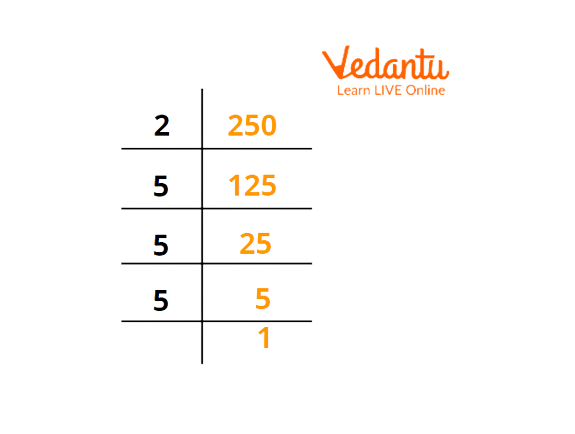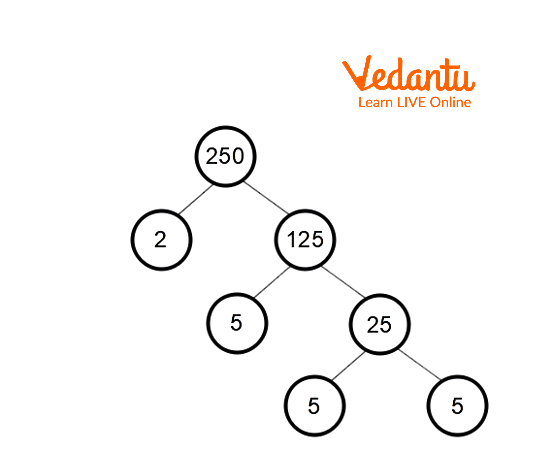Courses
Courses for Kids
Free study material
Free LIVE classes
More

# Methods to Find Factors of 250LIVE
Join Vedantu’s FREE Mastercalss

## Introduction to Factors of 250

The numbers that when multiplied together, result in the number 250 are called factors of 250. For instance, when we multiply 2 and 5, we get 10, which means that 2 and 5 are factors of 10. The factors of the number 250 may be discovered in the same way. You will learn the factors of 250, how to discover the factors of a number 250, and the prime factors of 250 in this article, along with clear instructions.

## What are the Factors of 250?

A factor of an integer is another integer that can divide the integer without leaving any remainder. So, the factors of 250 are the numbers that divide 250 exactly without a remainder. Hence, all factors of 250 are 1, 2, 5, 10, 25, 50, 125 and 250.

## How Can We Find the Factors for 250?

To calculate the factor of 250, just divide 250 by all the integers from 1 to 250. Also, find the integer that divides 250 without leaving a remainder. Let's do it and figure out all the 250 factors.

1 is a factor of 250.

$250\div 1=250$

2 is a factor of 250.

$250\div 2=125$

5 is a factor of 250.

$250\div 5=50$

10 is a factor of 250.

$250\div 10=25$

25 is a factor of 250.

$250\div 25=10$

50 is a factor of 250.

$250\div 50=5$

125 is a factor of 250.

$250\div 125=2$

250 is a factor of 250.

$250\div 250=1$

Thus, the factors of 250 are 1, 2, 5, 10, 25, 50, 125, and 250.

## Prime Factorization of 250

The set of prime numbers whose product is 250 is known as the prime factorization of the number 250.

Divide 250 by the smallest prime number possible to get the prime factor of 250. Then, take the result and divide it by the lowest prime number. Repeat this step until we have 1.

The first step is to divide 250 by the lowest prime integer, which is 2.

$250\div 2=125$

Divide 125 by 5 because 125 is multiples of 5.

$125\div 5=25$

Again divide 25 by 5

$25\div 5=5$

Divide 5 by 5
$5\div 5=1$

Thus, the prime factorization of 250 is $2\times 5\times 5\times 5$ or $2\times {{5}^{3}}$, where 2 and 5 are prime numbers.Prime Factorization of 250

## Prime Factor Tree of 250

Factor trees represent the factors of a number, especially its prime factorization. Each tree branch is divided into factors. The end of the branch in the factor tree must be a prime number because the only two factors are itself and one, so the branch stops.Factor Tree of 250

## Factors of 250 Pairs

Pair factors may be positive or negative, but they cannot be a fraction or a decimal. To get the pair factor of 250, multiply the two numbers in a pair to obtain the original number, which is 250, as follows:

### Positive Factors of250

Factor Pair of 250

$1\times 250=250$

$\left( 1,\text{ }125 \right)$

$2\times 125=250$

$\left( 2,\text{ }125 \right)$

$5\times 50=250$

$\left( 5,\text{ }50 \right)$

$10\times 25=250$

$\left( 10,\text{ }25 \right)$

So, the positive pair factors of 250 are $\left( 1,\text{ }125 \right),\text{ }\left( 2,\text{ }125 \right),\text{ }\left( 5,\text{ }50 \right)$ and $\left( 25,\text{ }10 \right)$.

### Negative Factors of250

Factor Pair of 250

$-1\times -250=250$

$\left( -1,\text{ }-125 \right)$

$-2\times -125=250$

$\left( -2,\text{ }-125 \right)$

$-5\times -50=250$

$\left( -5,\text{ }-50 \right)$

$-10\times -25=250$

$\left( -10,\text{ }-25 \right)$

So, the negative pair factors are $\left( -1,\text{ }-125 \right),\text{ }\left( -2,\text{ }-125 \right),\text{ }\left( -5,\text{ }-50 \right)$ and $\left( -10,\text{ }-25 \right)$.

## Interesting Facts

• 250 has a total of 8 factors. The total of all 250 factors equals 468.

• Remember that 1 is not a prime number and, thus, it will not appear in any factor tree.

## Solved Important Questions

1. Find common factors of 150 and 250.

Ans: A factor is an integer that is divided by the original number into equal parts without the remainder. A number that divides each of the provided numbers perfectly is a common factor of two or more numbers.

Hence, factors of 150 and 250 are 1, 2, 3, 5, 6, 10, 15, 25, 30, 50, 75, and 150, and 1, 2, 5, 10, 25, 50, 125, and 250, respectively.

Therefore, the common factors of 150 and 250 are 1, 2, 5, 10, 25, and 50.

2. What are the factors of 52?

Ans: The factors of 52 are the numbers that divide 52 exactly with no remainder. So,

$52\div 1=52$

1 is a factor of 52.

$52\div 2=26$

2 is a factor of 52.

4 is a factor of 52.

$52\div 4=13$

13 is a factor of 52.

$52\div 26=2$

2 is a factor of 52.

$52\div 52=1$

52 is a factor of 52.

Therefore, the factors of 52 are 1, 2, 4, 13, 26, and 52.

3. Draw a factor tree of 33.

Solution: Factor trees represent the factors of a number, especially its prime factorization.

Thus, prime factors of 33 are $3\times 11$.Factor Tree of 33

## Conclusion

The numbers that divide 250 exactly with no remainder are known as the factors of 250. The factors of 250 are 1, 2, 5, 10, 25, 50, 125 and 250 and the prime factor of 250 is 2 5 5 5 or 2 53, where 2 and 5 are prime numbers. The positive pair factors of 250 are (1, 125), (2, 125), (5, 50) and (25, 10) and the negative pair factors are (-1, -125), (-2, -125), ( -5, -50) and (-25, -10).

## Practice Questions

1. How many factors does 250 have?

1. 5

2. 9

3. 8

4. 3

Ans: Option C

2. Is 250 a composite number?

1. Yes

2. No

Ans: Option A

Last updated date: 18th Sep 2023
Total views: 110.7k
Views today: 3.10k

## FAQs on Methods to Find Factors of 250

1. Write all the factors of 9.

The numbers that divide 9 exactly with no remainder are known as the factors of 9. So, the factors of 9 are 1, 3, and 9 and the prime factorization of 9 is 3 or 32, where 3 is a prime number. The pair factors of 9 are (1, 9), (3, 3), (-1, -9) and (-3, -3).

2. How to find the prime factors of 52?

Prime factorization of 52 refers to the method of determining the prime factors of 52. Divide 52 by the smallest prime number possible to get the prime factor of 52. Then, take the result and divide it by the lowest prime number. Repeat this step until we have 1.

Step 1: Divide 52 by 2

$52\div 2=26$

Step 2: Again divide 26 by 2

$26\div 2=13$

Step 3:  Divide 13 by 13

$13\div 13=1$

Thus, we get 1 at the end of this division method, and we can’t go on with the division approach. Hence, the prime factor of 52 is $2\times 2\times 13$ or $13\times {{2}^{2}}$, where 2 and 13 are prime numbers.

3. What will be the factors of 529, explained with help of a factor tree.

Factor trees represent the factors of a number, especially its prime factorization. Each tree branch is divided into factors. The end of the branch in the factor tree must be a prime number because the only two factors are itself and one, so the branch stops.

Thus, the prime factors of 529 are $23\times 23$